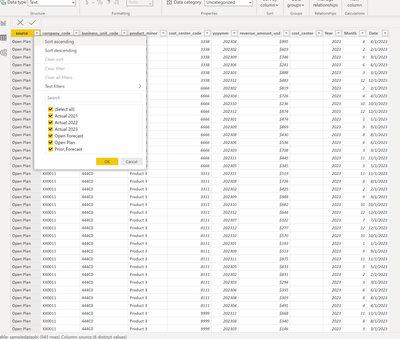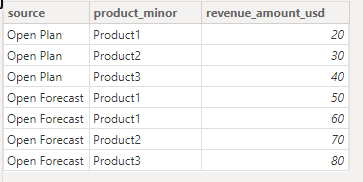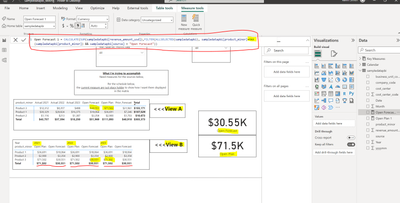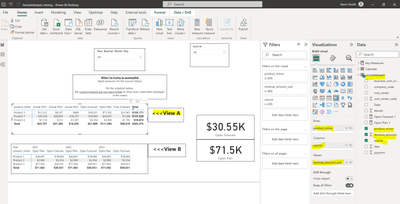cancel
Showing results for
Did you mean:Resolver I

## Dax measure need for matrix

PBI Community Good!

What I'm trying to accomplish

Need measures for the sources in my matrix in the screen shots below

I have a sample data set to forward if needed please advise.

Per the schedule below,

the current measure are just place holder to show how I want them displayed in the matrixThank You!

1 ACCEPTED SOLUTIONCommunity Support

Hi @PBI-Curious ,We can create measures.

``````Open Plan =
CALCULATE(SUM('Table'[revenue_amount_usd]),FILTER(ALLSELECTED('Table'),'Table'[product_minor]=SELECTEDVALUE('Table'[product_minor]) && 'Table'[source]="Open Plan" && 'Table'[date]=SELECTEDVALUE('Table'[date])))``````
``````Open Forecast =
CALCULATE(SUM('Table'[revenue_amount_usd]),FILTER(ALLSELECTED('Table'),'Table'[product_minor]=SELECTEDVALUE('Table'[product_minor]) && 'Table'[source]="Open Forecast" && 'Table'[date]=SELECTEDVALUE('Table'[date])))``````
``````total open forecast = SUMX(

SUMMARIZE(

'Table',

'Table'[product_minor],
'Table'[date],

"total",'Table'[Open Forecast]
),

[total]

)``````
``````total open plan = SUMX(

SUMMARIZE(

'Table',

'Table'[product_minor],
'Table'[date],

"total",'Table'[Open Plan]
),

[total]

)``````If the above one can't help you get the desired result, please provide some sample data in your tables (exclude sensitive data) with Text format and your expected result with backend logic and special examples. It is better if you can share a simplified pbix file. Thank you.

Refer to:

How to provide sample data in the Power BI Forum

Best Regards,

Neeko Tang

If this post  helps, then please consider Accept it as the solution  to help the other members find it more quickly.

7 REPLIES 7Resolver I

Hello Tang

Per your solution above, I got the measure to work.

Much appreciate your time and help!

Thank youResolver I

Hello Tang

Good Day!

Did you receive the link to my sample.pbix sent to you via a private message?Resolver I

Hello Neeko Tang

Per your example, I working on it now, but I'm getting an error message in the Dax.

I have a sample .pbix I can upload the same way for your review.

Thank youCommunity Support

Hi @PBI-Curious ,

According to your description, here are my steps you can follow as a solution.

(1) This is my test data.(2) We can create measures.

``Open Forecast = CALCULATE(SUM('Table'[revenue_amount_usd]),FILTER(ALLSELECTED('Table'),'Table'[product_minor]=MAX('Table'[product_minor]) && 'Table'[source]="Open Forecast"))``
``Open Plan = CALCULATE(SUM('Table'[revenue_amount_usd]),FILTER(ALLSELECTED('Table'),'Table'[product_minor]=MAX('Table'[product_minor]) && 'Table'[source]="Open Plan"))``

(3) Then the result is as follows.Or  we can create measures.

``````_a = SWITCH(TRUE(),
MAX('Table'[source])="Open Forecast",CALCULATE(SUM('Table'[revenue_amount_usd]),FILTER(ALLSELECTED('Table'),'Table'[product_minor]=MAX('Table'[product_minor]) && 'Table'[source]="Open Forecast")),
MAX('Table'[source])="Open Plan",CALCULATE(SUM('Table'[revenue_amount_usd]),FILTER(ALLSELECTED('Table'),'Table'[product_minor]=MAX('Table'[product_minor]) && 'Table'[source]="Open Plan")))``````
``````Value =

SUMX(

SUMMARIZE(

'Table',

'Table'[product_minor],
'Table'[source],

"total",'Table'[_a]
),

[total]

)``````Best Regards,

Neeko Tang

If this post  helps, then please consider Accept it as the solution  to help the other members find it more quickly.Resolver I

Hello Neeko Tang

I have questions per the following:

<<<<<<<<View B>>>>>

1. I got the Dax to work, but my totals for all product are incorrect .
2.  Not sure if I understood the reason for the =Max in the Dax Measure - Open Forecast 1 circled in red.
3. My cards for Open Forecast and Open Plan are showing the max value for Product 3 and not the totals. Also the totals match the max values.
4. All the numbers are the same for each year and category in the Matrix underlined in red.Visualizations details for Matrix <<<View AThank youCommunity Support

Hi @PBI-Curious ,We can create measures.

``````Open Plan =
CALCULATE(SUM('Table'[revenue_amount_usd]),FILTER(ALLSELECTED('Table'),'Table'[product_minor]=SELECTEDVALUE('Table'[product_minor]) && 'Table'[source]="Open Plan" && 'Table'[date]=SELECTEDVALUE('Table'[date])))``````
``````Open Forecast =
CALCULATE(SUM('Table'[revenue_amount_usd]),FILTER(ALLSELECTED('Table'),'Table'[product_minor]=SELECTEDVALUE('Table'[product_minor]) && 'Table'[source]="Open Forecast" && 'Table'[date]=SELECTEDVALUE('Table'[date])))``````
``````total open forecast = SUMX(

SUMMARIZE(

'Table',

'Table'[product_minor],
'Table'[date],

"total",'Table'[Open Forecast]
),

[total]

)``````
``````total open plan = SUMX(

SUMMARIZE(

'Table',

'Table'[product_minor],
'Table'[date],

"total",'Table'[Open Plan]
),

[total]

)``````If the above one can't help you get the desired result, please provide some sample data in your tables (exclude sensitive data) with Text format and your expected result with backend logic and special examples. It is better if you can share a simplified pbix file. Thank you.

Refer to:

How to provide sample data in the Power BI Forum

Best Regards,

Neeko Tang

If this post  helps, then please consider Accept it as the solution  to help the other members find it more quickly.Resolver I

Hello Tang

I sent you a private message with an sample.pbix.

I will take a look at your post above.